Importance and Contribution

The outputs described in this section are likely to be phased out in future versions of Q. Please refer to Driver (Importance) Analysis for the newer methods for computing importance.

Importance is an estimate of the sensitivity of the dependent question to changes in the independent questions (or, to use the language more commonly associated with regression, the independent variables and the dependent variable). For example, if a regression model reveals that the effect of a small change in price on sales is twice the effect of a similarly small change in advertising, then the importance of price will be computed as twice that of advertising.

Contribution is a calculation of the extent to which an independent question explains variation in the dependent question in the data. Whereas importance identifies differences in sensitivity, contribution is also influenced by the extent of variation of the independent variables. For example, price may be twice as important as advertising, but the contribution of the advertising can be greater than that of price if the data contains lots of variation in advertising but little in price (i.e., if the data shows little difference in price, then price cannot have had a large contribution to the dependent variable, sales).

Importance can be thought of as a measure of the potential of variables, whereas contribution is more of a measure of the historical impact of the variables.

Please note: there is no standard method for computing importance and contribution. Both are ill-defined concepts with no well-defined properties. They should be used only as rough guides and even then with caution.

Importance

In Q, importance is defined as the absolute value of the coefficients normalized to add to 1.0. More formally, where linear regression estimates the coefficient,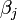$\beta_j$ for the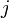$j$th of$J$ independent variables, where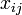$x_{ij}$ is the$i$ of$I$ observations on the$j$ variable,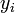$y_i$ is the$i$th observed value of the dependent variable,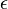$\epsilon$ is an error term, and the model is of the form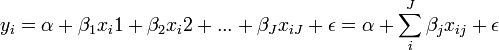$y_i = \alpha + \beta_1 x_i1 + \beta_2 x_i2 + ... + \beta_J x_{iJ} + \epsilon = \alpha + \sum_i^J \beta_{j} x_{ij}+ \epsilon$, the importance of the$j$th variable,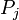$P_j$ is defined as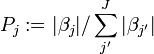$P_j := |\beta_j| / \sum^J_{j'}{|\beta_{j'}}|$.

For example, if two coefficients are estimated as 7 and -3, their importances are, respectively,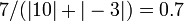$7/(|10| + |-3|)=0.7$ and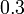$0.3$

This definition of importance implicitly assumes that the independent variables have been measured on similar scales (e.g., each independent variable containing data on a scale of 1 to 7). Where this assumption is inappropriate, it may be appropriate to instead compute importance using the formula above with the Beta scores shown in the outputs of the regression.

Where a question contains multiple variables,$\beta_j$ is replaced by the greater of 0 and the largest coefficient less the smaller of 0 and the smallest coefficient (which means that models comparing categorical to continuous variables will likely over-state the impact of the categorical variable in small samples). Please note: this calculation is designed for situations where the question is categorical or contains a numeric variable with categorical coding of special effects (e.g., missing data). Where the question contains multiple numeric variables, the estimated importance of their combined effect will be downwards-biased (i.e., if wanting to estimate the cumulative importance of multiple variables, each should be used as a separate question in the model and then their computed importances summed at the end).

Contribution

Contribution is an estimate of the impact that an independent question (variable) is observed to have had on the dependent variable in the data.

Q's computations assume that the dependent variable is numeric (even when it is binary or ordered).

Where$\beta_j$ is the coefficient of the$j$th of$J$ independent variables, and where$x_{ij}$ is the$i$ of$I$ observations on the$j$ variable, the contribution of the$j$th variable,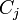$C_j$ is defined as: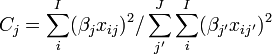$C_j = \sum_i^I (\beta_{j} x_{ij} )^2 /\sum_{j'}^J\sum_i^I(\beta_{j'} x_{ij'} )^2$

For example, with two coefficient estimated as 7 and -3, the respective independent variables and resulting calculations of contribution are shown in the table below.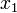$x_1$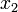$x_2$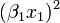$(\beta_1 x_1)^2$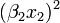$(\beta_2 x_2)^2$
1 1$(7 \times 1)^2 =$49 9
2 1 196 9
1 2 49 36
2 2 196 36
Total 490 90
Contribution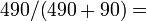$490/(490+90)=$0.84 0.16

The contribution scores are scale-independent (i.e., transforming the independent variables by modifying their variance will not change the computed contribution scores).

Further reading: Key Driver Analysis Software